# Shape Construction - Non Verbal Reasoning questions

## Shape Construction - Non Verbal Reasoning questions

Q1. Select the alternative which represents three out of the five alternative figures which when fitted into each other would form a complete square.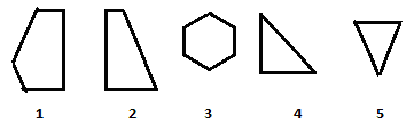A. 123
B. 234
C. 125
D. 124

Q2. Select the alternative which represents three out of the five alternative figures which when fitted into each other would form a complete square.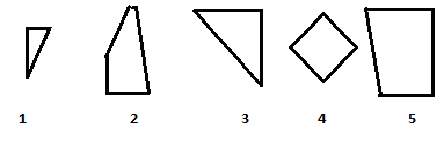A. 125
B. 234
C. 143
D. 145

Q3. Select the alternative which represents three out of the five alternative figures which when fitted into each other would form a complete rectangle.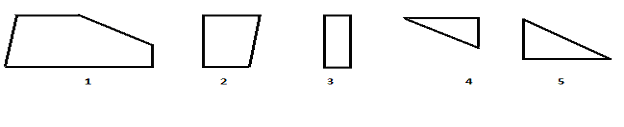A. 123
B. 421
C. 234
D. None of these

Q4. Select the alternative which represents three out of the five alternative figures which when fitted into each other would form a complete rectangle.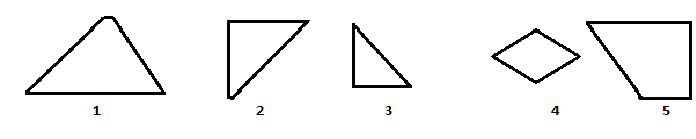A. 231
B. 215
C. 124
D. 125

Q5. Select the alternative which represents three out of the five alternative figures which when fitted into each other would form a complete rectangle.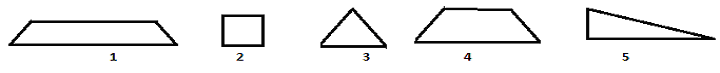A. 123
B. 315
C. 413
D. 543

Q6. Select the alternative which represents three out of the five alternative figures which when fitted into each other would form a complete Square.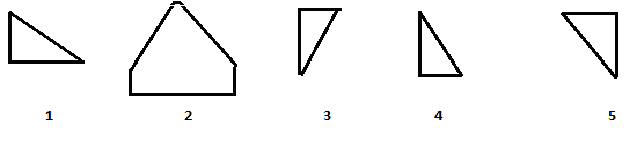A. 123
B.325
C. 135
D. 234

Q7. Select the alternative which represents three out of the five alternative figures which when fitted into each other would form a complete Square.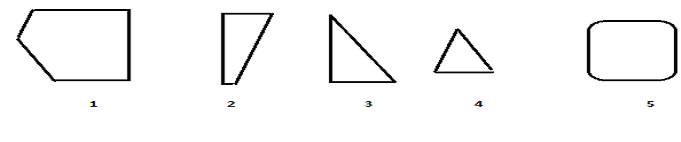A. 123
B.124
C.125
D. 234

Q8. Select the alternative which represents three out of the five alternative figures which when fitted into each other would form a complete Triangle.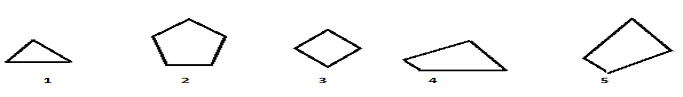A. 123
B.234
C.145
D. None of these

Q9. Select the alternative which represents three out of the five alternative figures which when fitted into each other would form a complete Circle.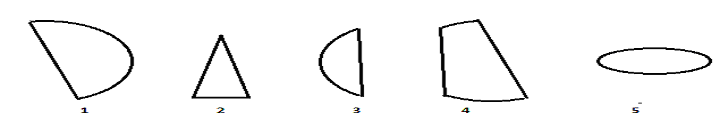A. 123
B. 134
C. 145
D. 135

Q10. Select the alternative which represents three out of the five alternative figures which when fitted into each other would form a complete Hexagon.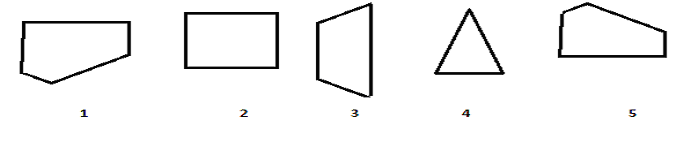A. 135
B.123
C.134
D. None of these

Q11. Select the alternative which represents three out of the five alternative figures which when fitted into each other would form a complete Pentagon.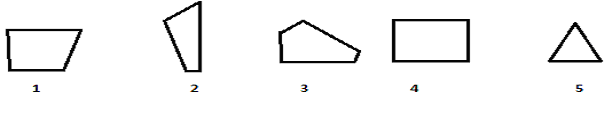A. 123
B.134
C.135
D.235

Q12. Select the alternative which represents three out of the five alternative figures which when fitted into each other would form a complete Diamond.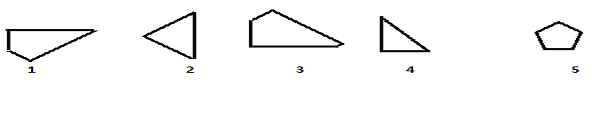A. 234
B. 123
C. 345
D. 134

Q13. Select the alternative which represents three out of the five alternative figures which when fitted into each other would form a complete Circle.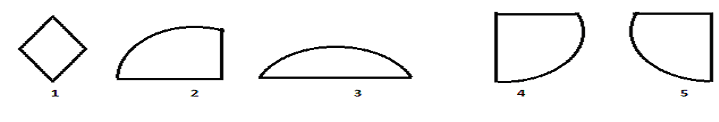A. 123
B. 234
C. 345
D. 134

Q14. Select the alternative which represents three out of the five alternative figures which when fitted into each other would form a complete Hexagon.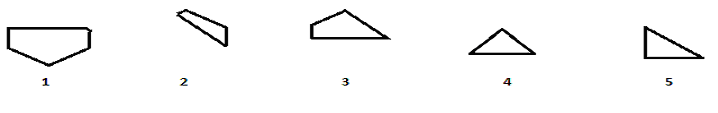A. 134
B.123
C. 345
D. 234

Q15. Select the alternative which represents three out of the five alternative figures which when fitted into each other would form a complete Pentagon.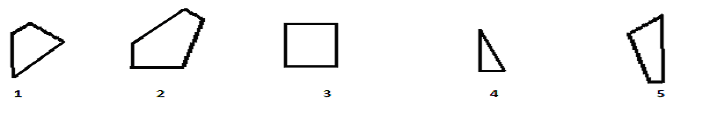A. 123
B. 125
C. 234
D.235

Q16. Select the alternative which represents three out of the five alternative figures which when fitted into each other would form a complete Ellipse.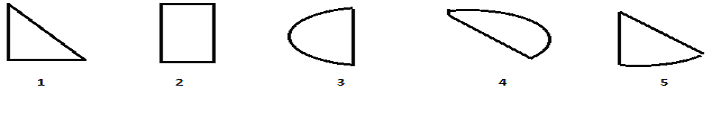A. 123
B. 134
C.342
D.345

Q17. Select the alternative which represents three out of the five alternative figures which when fitted into each other would form a complete Rounded Rectangle.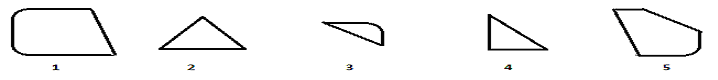A. 123
B. 134
C. 234
D. 135

Q18. Select the alternative which represents three out of the five alternative figures which when fitted into each other would form a complete Triangle.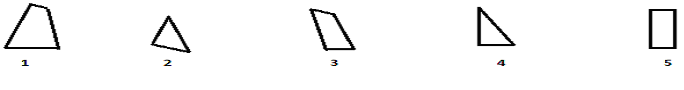A. 123
B.234
C.345
D.245

Q19. Select the alternative which represents three out of the five alternative figures which when fitted into each other would form a complete Triangle.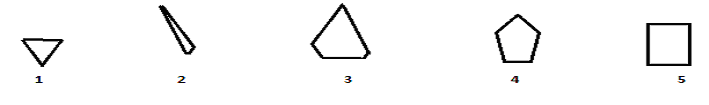A. 123
B. 134
C. 345
D. 234

Q20. Select the alternative which represents three out of the five alternative figures which when fitted into each other would form a complete Right Angle Triangle.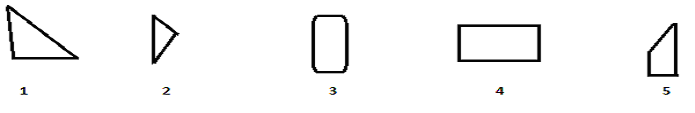A. 123
B. 134
C. 234
D. None of these

Q21. Select the alternative which represents three out of the five alternative figures which when fitted into each other would form a complete Pentagon.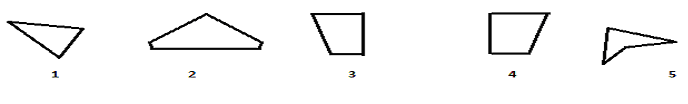A. 234
B. 123
C. 325
D. None of these